Next: Inverse Compton Scattering Up: ph_rad Previous: Photoelectric Absorption

# Compton Scattering

In the Thomson scattering, the classical picture of the photon-free-electron scattering, there would be no change of energy of the electromagnetic field. In other word, the frequency/wavelength of light should maintain same before and after the scattering. In 1924, Compton observed scattering between X-ray and electrons where wavelength of the scattered light becomes larger than its initial value by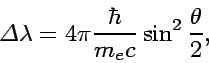(20)

which appears to be inconsistent with the classical theory.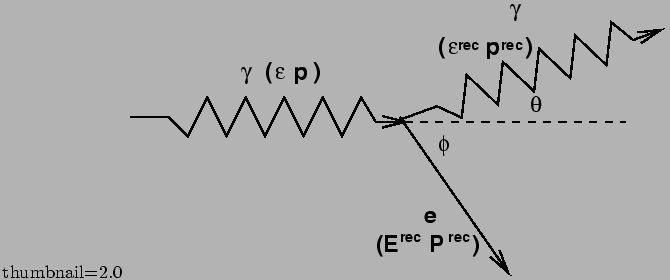This apparent inconsistency turned out to be understood in the Quantum picture where the radiation field is equivalent to a photon. In the system illustrated in FIG. 4, The energy momentum conservation leads to the following equations: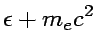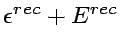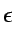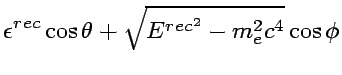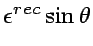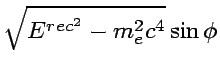(21)

Finally we get the recoiling photon energy as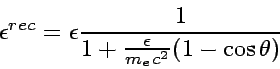(22)

and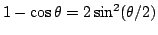indeed gives Eq. 20.

It should be remarked that the recoiling energy given by Eq. 22 can be easily obtained by the 4-momentum treatment in the scheme of the relativistic kinematics. The photon 4-momentum vector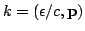and the electron 4-momentum vector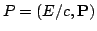follows the conservation law in the collision such as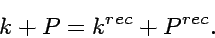(23)

Then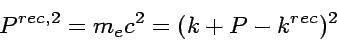(24)

and using that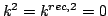and that the momentum component of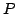vanishes as the initial electron is at rest leads to Eq. 22.

The cross section of the Compton Scattering is described by the Kline-Nishina Formula: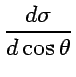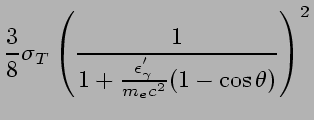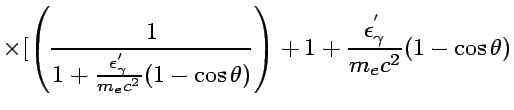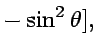(25)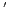implies the valuables described in the electron rest system.

SubsectionsNext: Inverse Compton Scattering Up: ph_rad Previous: Photoelectric Absorption
Shigeru Yoshida
2002-07-18